Contents - Index

Uncertainty Propagation

In many cases, an important quantity is not directly measured by rather calculated as a function of one or more variables that are directly measured, i.e., Y = f( X1, X2, ....).  The measured variables, X1, X2, etc. have with a random variability which is referred to as its uncertainty.  In EES, that uncertainty is displayed with a ± symbol, e.g., X1 = 300 ± 2.

The purpose of this command is to calculate how the uncertainties in each of the measured variables propagate into the value of the calculated quantity, Y.   The method for determining this uncertainty propagation is described in NIST Technical Note 1297 (Taylor B.N. and Kuyatt, C.E., Guidelines for Evaluating and Expressing the Uncertainty of NIST Measurement Results, National Institute of Standards and Technology Technical Note 1297, 1994).  Assuming the individual measurements are uncorrelated and random, the uncertainty in the calculated quantity can be determined as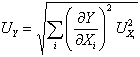where U represents the uncertainty of the variable.

After selecting this command, EES will present two lists of variables.  Select one or more variables (up to 12 in the Commercial version and 50 in the Professional version) for which the uncertainty propagation is to be determined from the list on the left.  Select one or more Measured variables from the list on the right.  The maximum number of Measured variables is 30 for the Commercial version and 200 for the Professional version.  To specify the uncertainty associated with the Measured variables, click the Set Uncertainties button below the right list.  A second dialog window will appear in which the absolute or relative (fraction of the measured value) uncertainties for each selected measured variable can be specified.  An uncertainty value for each measured variable must be provided.  Note that the uncertainty value can be supplied using another variable.  Click the OK button to set the uncertainties and close the Specify Uncertainties dialog window.  Click the OK button Uncertainty Propagation dialog to start the calculations.

Example:

"!Use the Uncertainty Propagation command (F6) in the  Calculate menu to calculate the uncertainty associated with the calculated heat transfer rate as a result of uncertainties in the measurements of T_infinity, T_s, and Vel."

Fluid\$='Air' {Define the fluid using a string variable}

L=0.5 [m]                  "Length of plate in flow direction in m"

Vel=20 [m/s]               "air velocity with an uncertainty of 10% of its value"

T_infinity=300 [°C]               "Air temperature in +/- 1 °C"

T_s=27 [°C]                        "Plate surface temperature +/- 1 °C"

Q=h*L*(T_infinity-T_s)      "heat transfer rate per unit width in W/m"

T_film=(T_infinity+T_s)/2      "Film temperature for evaluating properties in °C"

rho=density(Fluid\$,T=T_film,P=100 [kPa])   "Air density in kg/m^3"

k=conductivity(Fluid\$,T=T_film) "Thermal conductivity in W/m-K"

mu=viscosity(Fluid\$,T=T_film) "Viscosity in kg/m-s"

Pr=Prandtl(Fluid\$,T=T_film) "Prandtl number"

Re=rho*Vel*L/mu "Reynold's number"

Nu#=0.664*Re^0.5*Pr^0.333 "Nusselt number for laminar flow"

(h*L)/k=Nu# "Definition of Nusselt number to find h"

Select the  Uncertainty Propagation command  (F6).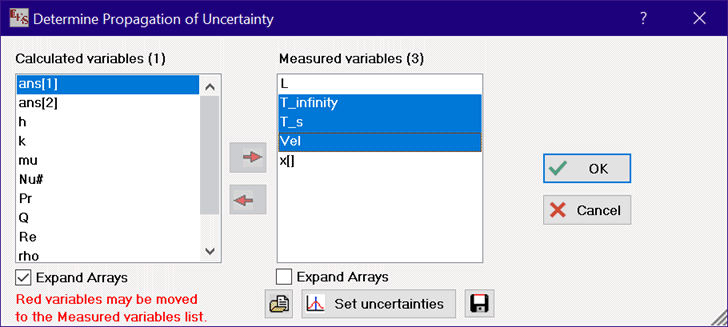Next, click the Set Uncertainties button and enter the uncertainty values for the Measured Variables.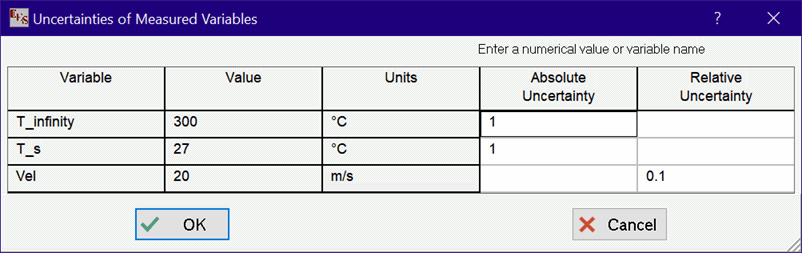The Professional version allows the uncertainty values to be entered in either the main or the alternate set of units.  Clicking on the up/down arrows in the units column toggles the display from the main set to the alternate set of units, which is shown in red, as seen in the example below.  If array variables are among the selected measured variables, the Professional version will provide the option to treat all of the variables in the array as one variable.  This option is invoked by unchecking the Show Array Variables check box at the upper left of the dialog. In the example below, specifying the uncertainty for x[] sets the uncertainty for all array elements in the x array that are in the measured variables list..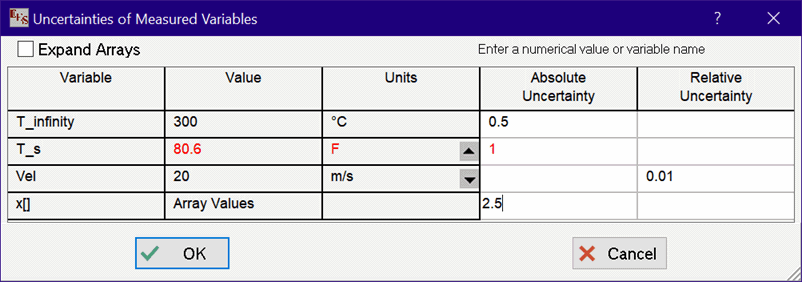Click OK for both dialogs to initiate the uncertainty calculations.  After the calculations are completed, EES will display an abbreviated Solution Window containing the calculated and measured variables and their respective uncertainties.  The uncertainty associated with the calculated variable can be assigned to another EES variable using the UncertaintyOf function.  The partial derivative of the calculated variable with respect to each measured variable will be displayed.  In addition the % of the total uncertainty in the calculated variable resulting from the uncertainty in each measured variable will also be displayed.  This % is calculated as the ratio of the square of the product of the partial derivative and uncertainty of each measured variable to the square of the uncertainty in the calculated variable.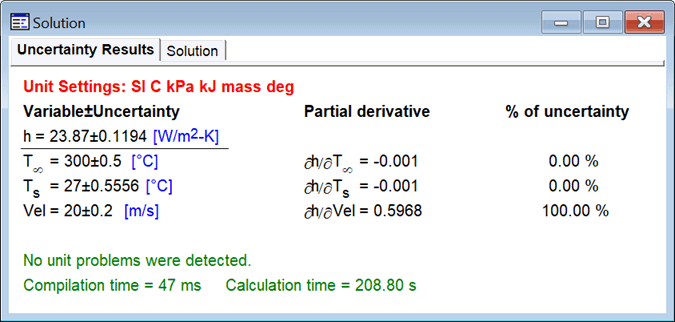Note that the format of the calculated variables, e.g., h and Q in the above figure, can be viewed or changed.  This is done by selecting the variable and either pressing the Enter key or double-clicking/right clicking the mouse.  (The Tab key can be used to move the focus to the variable that you wish to select.)  This action will bring up the Specify Format and Units dialog where the changes, such as the yellow highlighting, can be made.

In the Professional version, the choices made for the calculated and measured variables and their associated relative or absolute uncertainties can be saved by clicking the Save Uncertainty Information button located to the right of the Set Uncertainties button.  These choices can later be restored by clicking the Open Uncertainty Information button at the left of the Set Uncertainties button.  Saving the uncertainty information allows one EES file to solve several different uncertainty problems without having to reenter the uncertainty setting.  The Save Uncertainty Information saves a text file having an .UNC file name extension.  This file can be viewed or edited with a text editor such as Notepad.  The .UNC file can also be used in a different EES file.  Variables that in the file but do not appear in the Calculated or Measured variable lists are ignored.

The variables initially appearing in the Measured variables list are constants, i.e., values that are independent of the values of other variables.  Variables that are read directly from the Lookup table (with no conversions) are also considered to be constants.  However, some variables (shown in red) that initially appear in the Calculated variable list can be moved to the Measured variables list.  For example, consider the following simplistic set of equations.

Row=1

X=Lookup('Lookup 1',Row,1)*convert(J,kJ)

Y=Lookup('Lookup 1',Row,2)*convert(J,kJ)

Z=X^2+Y^3

In this case, X and Y are read from the first row of a Lookup table.  Z is a function of X and Y and the uncertainty propagation of X and Y into Z is to be determined.  Selecting the Uncertainty Propagation command produces the following display: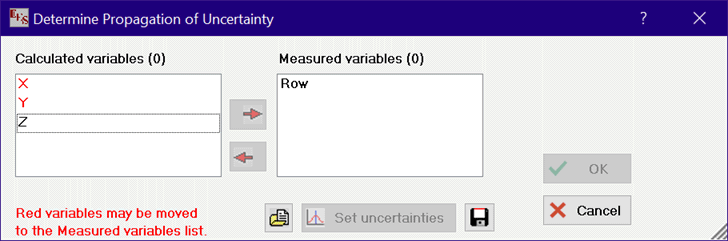Note that EES cannot unambiguously determine that X and Y are constants.  Row is the only variable initially appearing in the Measured variables list since it is the only variable that is completely independent of all other variables.  However, X and Y (which are both functions of Row) can be moved the Measured variables list by selecting each variable and then clicking the arrow button (or dragging the selection to the Measured variables list).  After this is done, the display will appear as: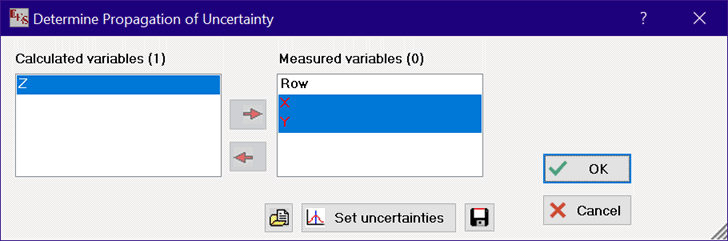and the uncertainty propagation of X and Y into Z can be determined as intended.

An alternative way to do this problem is to use the \$DOLAST directive. For example, consider the equations below:

Row=1

X=Lookup('Lookup 1',Row,1)*convert(J,kJ)

Y=Lookup('Lookup 1',Row,2)*convert(J,kJ)

\$DOLAST

Z=X^2+Y^3

\$ENDDOLAST

If a \$DOLAST directive is employed, EES will first attempt to solve the equations that are not contained within the \$DOLAST - \$ENDDOLAST clause.  After calculations are completed, the variables, e.g., X and Y, are set to constants.  Then the Uncertainty propagation calculations are initiated for the equations remaining in the \$DOLAST - \$ENDDOLAST clause.

See also:           Uncertainty Propagation Table

UncertaintyOf